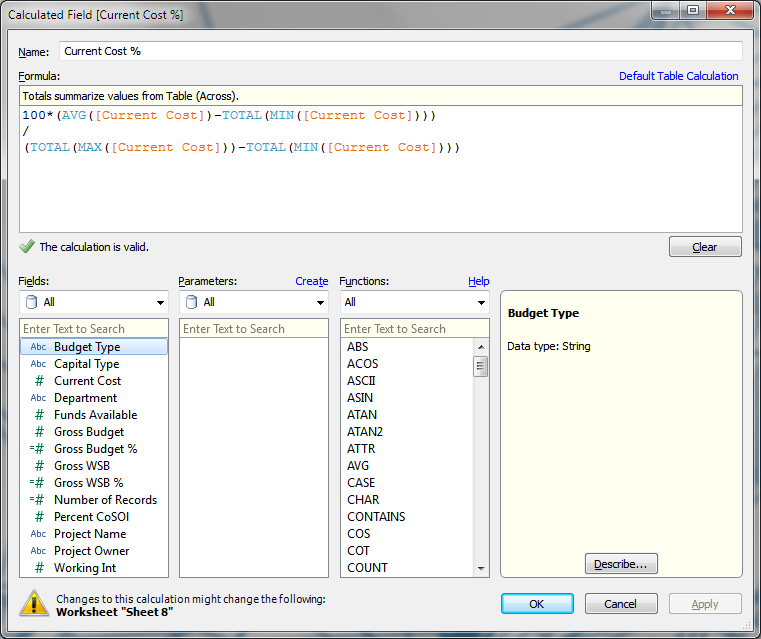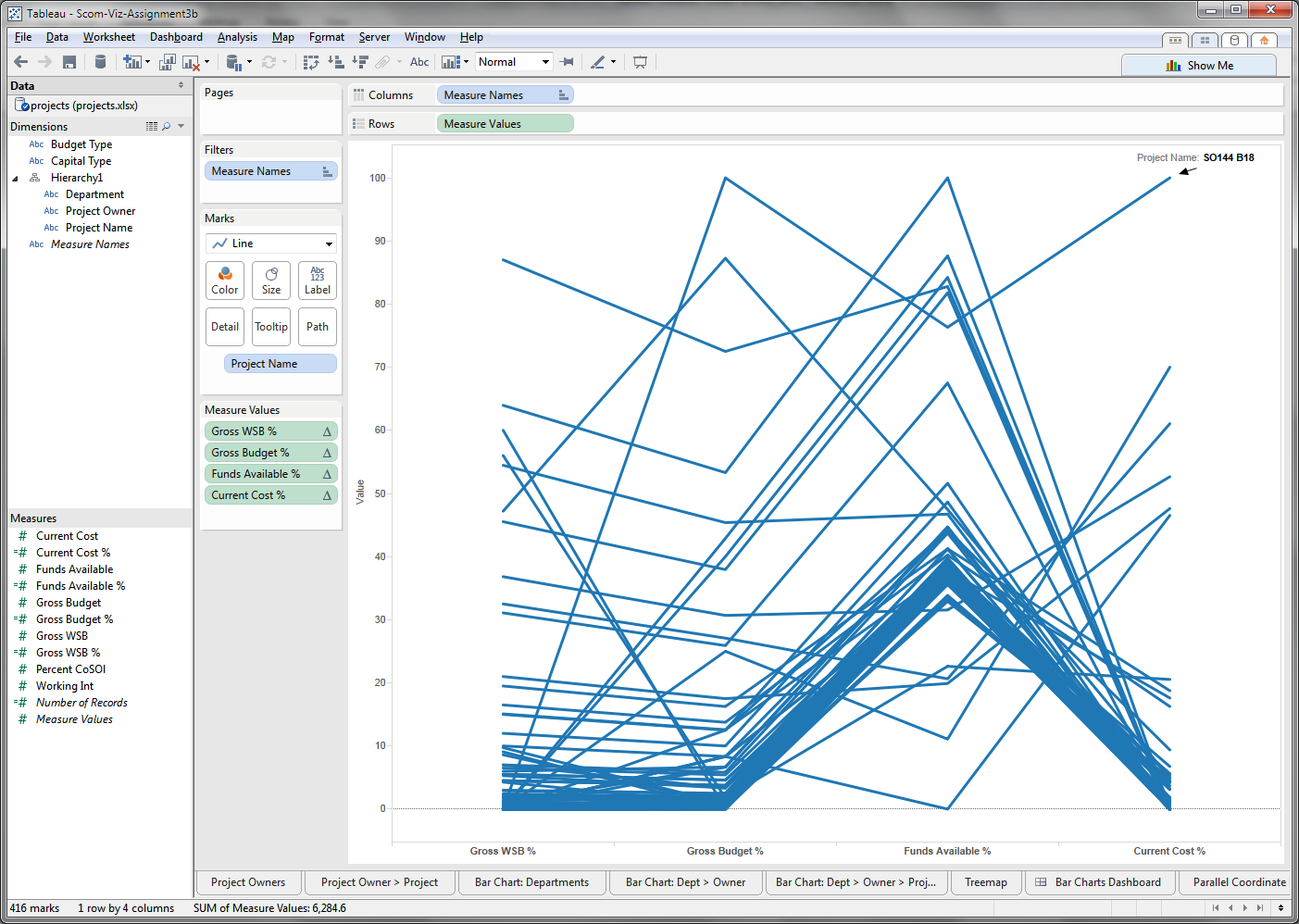## Wednesday, April 02, 2014

### Parallel coordinate plot in Tableau: a workaround

The parallel coordinate plot is useful for visualizing multivariate data in a dis-aggregated way, where we have multiple numerical measurements for each record. A scatter plot displays two measurements for each record by using the two axes. A parallel coordinate plot can display many measurements for each record, by using many (parallel) axes - one for each measurement.

While not as popular as other charts, it sometimes turns out to be useful, so it's good to have it in the visualization toolkit. Software such as TIBCO Spotfire and XLMiner include the parallel coordinate plot. There's even a free Excel add-on. But surprisingly, Tableau 8.1 still does not include this chart among its many neat options. After searching and not finding a straightforward answer, I found a simple workaround that allows creating a parallel coordinate plot in Tableau. For all those in search - here's the solution:

First, make sure to scale each measurement to a 0%-100% scale if it isn't already in that form (or if all the measurements on are the same scale, skip this step). In Tableau, scaling can be done by creating a new measure (Analysis> Create Calculated Field). The following formula will create a new scaled measure by subtracting the MIN and dividing by the range for a variable named Current Cost:

100*(AVG([Current Cost])-TOTAL(MIN([Current Cost]))) /
(TOTAL(MAX([Current Cost]))-TOTAL(MIN([Current Cost])))

Here's what it looks like in the Calculated Field menu:First scale each measurement to a o-100% scale by creating a new calculated field with this formula
Now create a parallel coordinate plot of the scaled measures:
1. Start a new worksheet
2. Drag Measure Names to the Columns shelf. Drag Measure Values to the Rows shelf.
3. In the Measure Values shelf you'll see all the measures. Keep only the scaled measures of interest and remove all other measures.
4. Change the bars to lines by selecting line in the Marks shelf.
5. Lastly, since we want each line to represent a single record, drag the appropriate Dimension (such as record ID) into the Detail shelf.

Voila! Here's an example of what the chart and the settings look like for four measurements (each line represents a single record, in this case, a project). Of course the beauty is then interacting with this chart: filtering, coloring, etc.Parallel coordinate plot implemented in Tableau
Thirsty for more? Check out the upcoming online course Interactive Data Visualization.